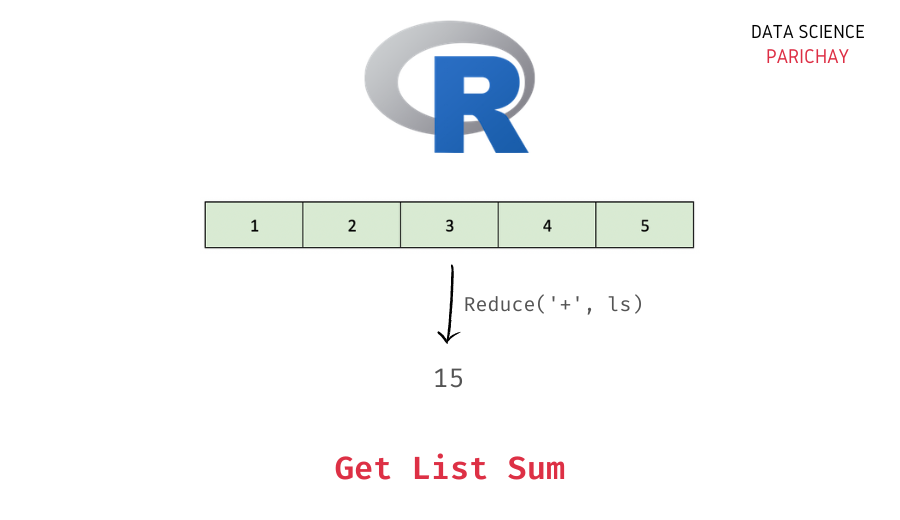# Sum of List Elements in R (Easy Examples)

In this tutorial, we will look at how to get the sum of elements in a list in R with the help of some examples.

## How to get the sum of values in a list in R?

You can use the `Reduce()` function to get the sum of values in an R list. Pass `'+'` as the first argument and the list as the second argument to the function. The following is the syntax –

```# sum of elements in list ls
Reduce('+', ls)```

The above function will compute the element-wise sum of each vector inside the list.

Note that scaler values are vectors on length one in R. So, if the list contains only scaler values, the resulting sum will also be scaler (that is, vector of length one). See the examples below.

## Examples

Let’s look at some examples of computing the sum of elements in a list.

### Sum of values in a list in R

First, let’s get the sum of a list of scaler values in R. For this, we will use the syntax mentioned above.

```# create a list
ls <- list(1, 2, 3)
# sum of list elements
print(Reduce('+', ls))```

Output:

` 6`

We get the sum of the list `list(1, 2, 3)` as 6 which is the correct answer (1+2+3 = 6).

📚 Data Science Programs By Skill Level

Introductory

Intermediate ⭐⭐⭐

🔎 Find Data Science Programs 👨‍💻 111,889 already enrolled

Disclaimer: Data Science Parichay is reader supported. When you purchase a course through a link on this site, we may earn a small commission at no additional cost to you. Earned commissions help support this website and its team of writers.

What happens if the list values are named? Let’s find out.

```# create a list with named elements
ls <- list("a"=1, "b"=2, "c"=3)
# sum of list elements
print(Reduce('+', ls))```

Output:

` 6`

Here, we create the same list as above but this time the elements are named. You can see that we get the same result as above, 6, for the sum of values in the list.

### Sum of a list of vectors in R

If the list has any vectors, the `Reduce()` function here will compute the element-wise vector sum. The following image illustrates how the sum gets calculated.

The resulting vector is of the same length as the individual vectors with each element being the corresponding sum of elements in the list vectors.

Let’s look at an example.

```# list with vector elements of same length
ls <- list(c(1, 2, 3), c(1, 1, 1))
# sum of list elements
print(Reduce('+', ls))```

Output:

` 2 3 4`

We get the element-wise sum of the individual vectors in the list.

If the vectors are of different lengths the shorter vector will be recycled to match the length of the longer vector. See the image below.

Let’s now look at an example.

```# list with vector elements of different lengths
ls <- list(c(1, 2, 3), c(1, 1))
# sum of list elements
print(Reduce('+', ls))```

Output:

```Warning message in f(init, x[[i]]):
“longer object length is not a multiple of shorter object length”
 2 3 4```

The resulting vector has the length of the longest vector in the list and is the sum of corresponding elements in the vectors. The shorter vector is duplicated to match the length of the longer vector for computing the sum.

You might also be interested in –

•